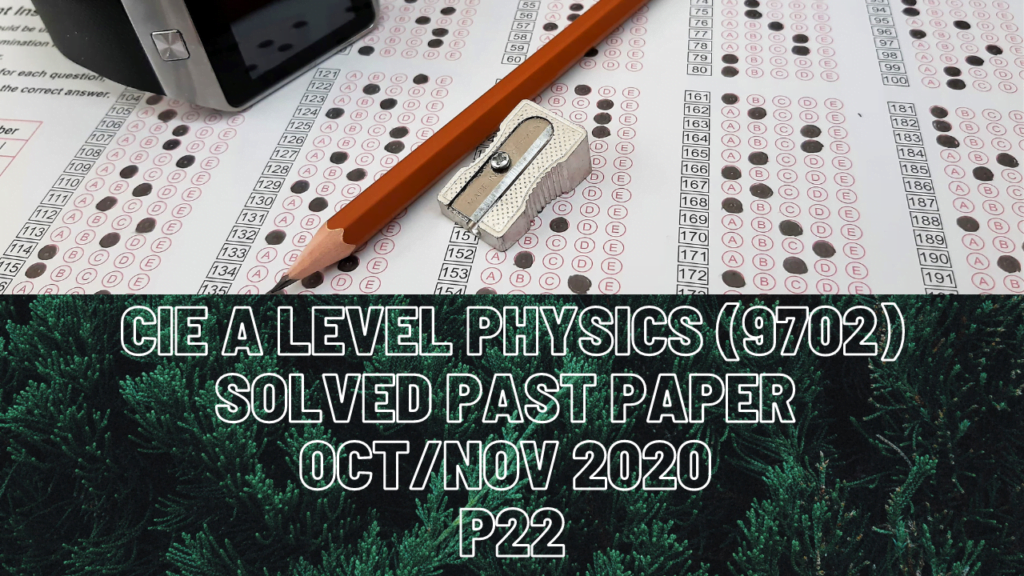A Level Physics### CIE A Level Physics Solved Past Paper Oct/Nov 2020 P22

1 bi) On distance versus time graph, when a slope gets steeper, the gradient increases and its acceleration. When the graph gets less steep, that denotes deceleration or decreasing gradient.

ii) For the part t=0 to t=1.5s, the train moves with decreasing speed and then travels at a constant speed for the next 1.5s. Speed is the gradient of distance versus time graph so the speed at t=2.0s is going to be the same as that at t=2.5s. To calculate speed from distance versus time graph, we take either the slope or tangent where the graph is a curve.

2 bi) Acceleration from speed versus time graph is its gradient which is the same for first 2.5s

3 bi) Elastic potential energy in the spring, when compression is 0.045m, is 0.81J and the kinetic energy of the ball when it’s released is 0.72J which means some energy was lost in the surroundings.

cii) Energy that was lost due to friction force is 0.72-0.60=0.12J

iii) Resultant force is directly proportional to acceleration. When there is air resistance while the ball rises up in air, resistie force and weight act downwards which make up the resultant force. When there is no air resistance, there is just ball’s weight that makes up the resultant force on the ball which is a lot smaller than resultant force when air resistance was significant. Hence acceleration in no air resistance is going to be smaller and time taken would be greater.

4b) The plank is in equilibrium thus total clockwise moment about end A will be equal to total anti-clockwise moment about the same point. Perpendicular forces to the pivot that rotate the plank anti-clockwise are vertical component of the plank’s weight and tension, T. Perpendicular force to the pivot that rotates the plank clockwise is 45N.

4 bi) A maximum forms only when the path difference between the two incoming waves is in whole number and a minimum forms when the path difference is in fractions. Path difference between the central maximum and its adjacent minimum on its either sides is always ?/2.

ii) Phase difference at minima is always 180 degrees

c) Intensity is directly proportional to amplitude squared so when amplitude doubles, intensity increases by four times.

6 bi) Current in the battery is sum of the currents in individual loops of parallel circuit.

ii) Following Kirchhoff’s second law, total EMF of the battery is equal to sum of the p.d.s across all components in the loop.

d) When resistance of Z decreases, combined resistance of the circuit also decreases. Resistance is directly proportional to voltage or p.d. thus total p.d. across the components decreases in this case.

7 biii) When the charge on the particle increases, electric force with which it is attracted also increases.